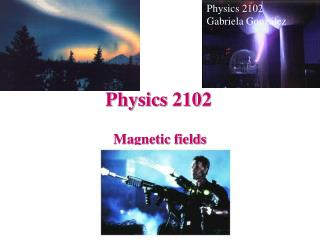DownloadDownload PresentationPhysics 2102

# Physics 2102

Download Presentation## Physics 2102

- - - - - - - - - - - - - - - - - - - - - - - - - - - E N D - - - - - - - - - - - - - - - - - - - - - - - - - - -
##### Presentation Transcript

1. Physics 2102 Gabriela González Physics 2102 Magnetic fields

2. A B v v Question Two charged ions A and B traveling with a constant velocity venter a box in which there is a uniform magnetic field directed out of the page. The subsequent paths are as shown. What can you conclude? • (a) Both ions are negatively charged. • (b) Ion A has a larger mass than B. • (c) Ion A has a larger charge than B. • (d) None of the above. FB= qvxB (a) F=qv x B. The vector v x B will point down when the charges enter the box; the force also points down for cw motion: charges must be positive. (b,c) r= mv/qBSame speed and B for both masses; larger radius for A than B. Ion with larger mass/charge ratio (m/q) moves in circle of larger radius. But that’s all we know! We cannot conclude b or c. (d) Is the right answer.

3. Crossed fields The figure shows four directions for the velocity vector v of a positively charged particle moving through a uniform electric field E (out of the page) and a uniform magnetic field B. • Rank directions 1, 2, 3 according to the magnitude of the net force on the particle. • If the net force is zero, what is the direction and magnitude of the particle’s velocity?

4. Electric and magnetic forces: example A solid metal cube moves with constant velocity v in the y-direction. There is a uniform magnetic field B in the z-direction. • What is the direction of the magnetic force on the electrons in the cube? • What is the direction of the electric field established by the electrons that moved due to the magnetic force? • Which cube face is at a lower electric potential due to the motion through the field? • What is the direction of the electric force on the electrons inside the cube? • If there is a balance between electric and magnetic forces, what is the potential difference between the cube faces (in terms of the cube’s velocity v, side length d and magnetic field B)?

5. Hot cathode emits electrons Get accelerated by positive plate Might be deflected using plates Produce point of light on screen. In a magnetic field: B v Fe Cathode ray tube (CRT) : TV, computer monitors before LCD Dot shifts sideways. http://en.wikipedia.org/wiki/Comparison_of_display_technology

6. L Magnetic force on a wire Note: If wire is not straight, compute force on differential elements and integrate:

7. L B i The Rail Gun rails • Conducting projectile of length 2cm, mass 1g carries constant current 10A between two rails. • Magnetic field B = 1T points outward. • Assuming the projectile starts from rest at t = 0, what is its speed after a time t = 1s? projectile • Force on projectile = iLB (from F = iLxB) • Acceleration = iLB/m (from F = ma) • v(t) = iLBt/m (from v = v0 + at) • = (10A)(0.02m)(1T)(1s)/(0.001kg) • = 200 m/s ~ 450 mph • But: d=0.5(iLB/m)t2 = 0.5 v(t) t = 100 m!!

8. Rail guns in the “Eraser” movie "Rail guns are hyper-velocity weapons that shoot aluminum or clay rounds at just below the speed of light. In our film, we've taken existing stealth technology one step further and given them an X-ray scope sighting system," notes director Russell. "These guns represent a whole new technology in weaponry that is still in its infancy, though a large-scale version exists in limited numbers on battleships and tanks. They have incredible range. They can pierce three-foot thick cement walls and then knock a canary off a tin can with absolute accuracy. In our film, one contractor has finally developed an assault-sized rail gun. We researched this quite a bit, and the technology is really just around the corner, which is one of the exciting parts of the story." Warner Bros., production notes, 1996. http://movies.warnerbros.com/eraser/cmp/prodnotes.html#tech Also: INSULTINGLY STUPID MOVIE PHYSICS: http://www.intuitor.com/moviephysics/